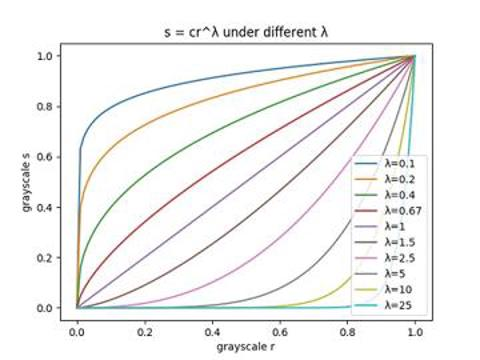# 数字图像处理/对比增强

## 幂次变换

``````s = T(r)
``````## 代码实现

``````import PIL.Image
import scipy.misc
import numpy as np

def convert_3d(r):
s = np.empty(r.shape, dtype=np.uint8)
for j in range(r.shape):
for i in range(r.shape):
s[j][i] = (r[j][i] / 255) ** 0.67 * 255
return s

im = PIL.Image.open('/img/jp.jpg')
im_mat = np.asarray(im)
im_converted_mat = convert_3d(im_mat)
im_converted = PIL.Image.fromarray(im_converted_mat)
im_converted.show()
``````

## 效果展示